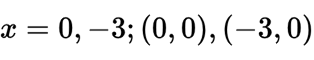Friday, October 30, 2020

How to find X and Y Intercept (Pre Calculus)

Problem Solutiony-intercept:x-intercept:y-intercept:x-intercept:None. y cannot equal 0y-intercept: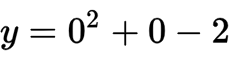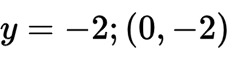x-intercept: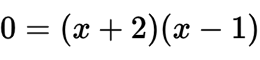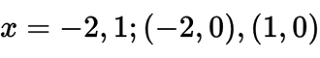y-intercept: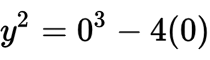x-intercept: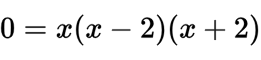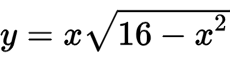y-intercept:x-intercept: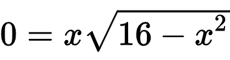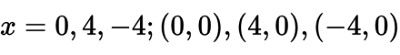y-intercept: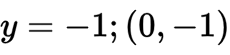x-intercept: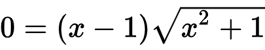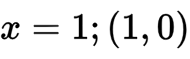y-intercept: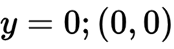x-intercept: